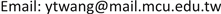1. 引言

  已在典型的两国模型中分析资本外移的福利效果，在其Heckscher-Ohlin 模型设定下，要素之报酬只是商品价格的函数，并不因要素本身数量的增减而改变，他证明，当出口商品是资本密集，资本外流是不利的。然而，这些传统文献并无法具体显现出经济整合之下同盟国彼此之间休戚相关、对外一致的特性，所以一些因经济结合而产生的效果将不免漏失。国际贸易理论一般认为关税同盟(customs union)是一些国家彼此结合，对于内部贸易不加任何限制，对外则设立一致的关税结构。由于生产要素开放迁移为经济整合的必经过程，欧体并且已于1993年实施，因此在经济整合之下探讨要素移动理论应将此特性列入考虑。但为了将分析焦点集中在资本流动之上，本文不拟讨论劳动迁徙的问题。在本文中，所将考虑的经济区域是一个允许资本自由移动的关税同盟。因此，会员国的资本出口将透过区域内的资本移动与共同对外设置的关税影响盟国的福利，此时会员国福利的改变将与传统分析有所区别。另外，整个区域与世界福利所受之影响亦有探讨的必要。在传统的Heckscher-Ohlin架构下，会员国的资本外流对整合区域内的资本市场不具影响，本文中会员国间资本自由移动的特性在此将无意义2。另一方面，鉴于外人直接投资经常发生在进口竞争产业，我们因此将资本限制在被投资之非会员国的进口部门，道种设定亦不乏文献采用，如   与  。基于这些目的，会员国的资本外流将被视为外生给定，此种讨论资本移动的方式亦为       与  等所采用，优点在于可清楚地看出直接由资本移动所引起的效果，这有别于藉由提高关税保护来考虑间接诱发的内生性资本移动效果    。另外，我们将组合  的特定要素(specific factor)模型架构与  的分析方式进行讨论。本文其余部份的结构如下：第二节建立我们的三国两商品三要素一般均衡模型。第三节首先讨论投资国、其盟国与整个经济区域由此投资获利或受损的情况；其次探讨会员国的投资是否必然有利于受投资之非会员国；最后，并就世界福利所受影响进行分析。第四节整理归纳本文的重要结果。

2. 基础模型

k a = k ¯ a − i − i c (1)

k b = k ¯ b + i (2)

k c = k ¯ c + i (3)

R = r k a ( p , k a ) = r k b ( p , k b ) (4)

e a ( p , u a ) = r a ( p , k a ) + ( 1 − ω ) r k c i c + i R + t ( e p a − r p a ) (5)

e b ( p , u b ) = r b ( p , k b ) − i R + t ( e p b − r p b ) (6)

e c ( p , u c ) = r c ( p , k c ) − ( 1 − ω ) r k c i c (7)

3. 结盟国资本外流之效益

d k j = ( r k k j r k k a + r k k b − 1 ) d i c ,     j = a , b (8)

e u a ( 1 − t c e a ) d u a = ( i r k k a − t r p k a ) d k a + [ ( 1 − ω ) r c k − R + ( 1 − ω ) i c r k k c ] d i c (9)

( 1 − ω ) ( r k c + i c r k k c ) = ( 1 − ω ) r k c ( 1 − ε c z )

e u b ( 1 − t c e b ) d u b = − ( i r k k b + t r p k b ) d k b (10)

[ ( 1 − ω ) r − k c r + ( 1 − ω ) i c r k k c ] d i c − t ( r p k a d k a + r p k b ) d k b (11)

e u c d u c = [ ω r k c − ( 1 − ω ) i c r k k c ] d i c (12)

e u c d u c = [ ω r k c ( 1 − ε c z ) − i c r k k c ] d i c

( r k c − R ) d i c − t ( r p k a d k a + r p k b d k b )

4. 结语